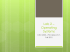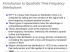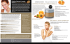# edz5 - University of York

## Transcription

edz5 - University of York
```Fast Jensen-Shannon Graph Kernel
Bai Lu and Edwin Hancock
Department of Computer Science
University of York
Supported by a Royal Society
Wolfson Research Merit Award
Structural Variations
Protein-Protein Interaction Networks
Manipulating graphs



Is structure similar (graph isomorphism,
inexact match)?
Is complexity similar (are graphs from
same class but different in detail)?
Is complexity (type of structure)
uniform?
Goals



Can we capture determine the similarity of structure
using measures that capture their intrinsic
complexity.
Can graph entropies be used for this purpose.
If they can then they lead naturally to information
theoretic kernels and description length for learning
over graph data.
Outline

Literature Review: State of the Art Graph Kernels
 Existing graph kernel methods：Graph kernels
based on a) walks, b) paths or c) subgraph or
subtree structures.
Prior Work: Recently we have developed on
information theoretic graph kernel based on
Jensen-Shannon divergence probability
distributions on graphs.
Fast Jensen-Shannon Graph Kernel:
 Based on depth depth-based subgraph
representation of a graph
 Based around graph centroid
Experiments
Conclusion




Literature Review: Graph Kernels

Existing Graph Kernels (i.e Graph Kernels from the Rconvolution [Haussler, 1999]) fall into three classes:
 Restricted subgraph or subtree kernels

Weisfeiler-Lehman subtree kernel [Shevashidze et al.,
2009, NIPS]

Random walk kernels
 Product graph kernels [Gartner et al., 2003, ICML]
 Marginalized kernels on graphs [Kashima et al., 2003,
ICML]

Path based kernels

Shortest path kernel [Borgwardt, 2005, ICDM]
Motivation

Limitations of existing graph kernel



Can not scale up to substructures of large size (e.g.
(sub)graphs with hundreds or even thousands vertices).
Compromised to substructures of limited size and only
roughly capture topological arrangement within a graph.
Even for relatively small subgraphs, most graph kernels still
Aim: develop a novel subgraph kernel for efficient
computation, even when a pair of fully sized
subgraphs are compared.
Approach

Investigate how to kernelize depth-based graph
representations by similarity for K-layer subgraphs
using the Jensen-Shannon divergence.



Commence by showing how to compute a fast JensenShannon diffusion kernel for a pair of (sub)graphs.
Describe how to compute a fast depth-based graph
representation., based on complexity of structure.
Combine ideas to compute fast Jensen-Shannon subgraph
kernel.
Notation

Notation
 Consider a graph

The vertex degree matrix of
is given by

Normalaised Laplacian and its spectrum
ˆ
ˆ
ˆT
Lˆ  D 1/ 2 ( D  A) D 1/ 2  
The Jensen-Shannon Diffusion Kernel

Jensen-Shannon diffusion kernel for graphs:

For graphs Gp and Gq, the Jensen-Shannon divergence is
where
is entropy of composite structure formed
from two (sub)graphs being compared (here we use the
disjoint union).

The Jensen-Shannon diffusion kernel for Gp and Gq is
where entropy H(·) is either Shannon or the von Neumann.
Composite Structure

Composite entropy of disjoint union

A disjoint union of a pair of graph of graphs Gp and Gq is
Graphs Gp and Gq are the connected components of the
disjoint union graph GDU.

Let p = |V p|/|V

Entropy (i.e. the composite entropy) of GDU is
DU
| and q = |V q|/|VDU|.
Graph Entropy: Measures of complexity

Shannon entropy of random walk : The probability of a
visiting vertex vi is
.
Shannon entropy of steady state random walk is

von Neumann entropy: entropy associated with normalised
Laplacian eigenvalues.
|V | ˆ
i ˆi
HV   ln
2
i 1 2
Approximated by (Han
PRL12)
Properties

The Jensen-Shannon diffusion kernel for graphs:


The Jensen-Shannon diffusion kernel is positive
definite (pd). This follows the definitions in [Kondor
and Lafferty, 2002, ICML], if a dissimilarity measure
between a pair of graphs Gp and Gq satisfies symmetry,
then a diffusion kernel associated with the similarity
measure is pd.
Time Complexity: For a pair of graphs Gp and Gq both
having n vertices, computing the Jensen-Shannon
diffusion kernel requires time complexity O(n^2).
Idea

Decompose graph into layered
subgraphs from centroid.

Use JSD to compare subgraphs.

Construct kernel over subgraphs.
The Depth-Based Representation of A Graph

Subgraphs from the Centroid Vertex

For graph G(V,E), construct shortest path matrix matrix SG whose
element SG(i, j) are the shortest path lengths between vertices vi
and vj . Average-shortest-path vector SV for G(V,E) is a vector with
element
from vertex vi to the remaining
vertices.

Centroid vertex for G(V,E) as

The K-layer centroid expansion subgraph
where
Depth-Based Representation

For a graph G, we obtain a family of centroid
expansion subgraphs
, the depthbased representation of G is defined as
where H(·) is either the Shannon entropy or the
von Neumann entropy.
Measures complexity via variation of entropy with
depth
The Depth-Based Representation

An example of the depth-based representation for a graph from the centroid vertex
Fast Jensen-Shannon Subgraph Kernel

For a pair of graphs Gp(Vp, Ep) and Gq(Vq, Eq), similarity measure is
is summed over an entropy-based similarity measure for the K-layer
subgraphs.

Jensen-Shannon diffusion kernel is the sum of the diffusion kernel
measures for all the pairs of K-layer subgraphs

Jensen-Shannon subgraph kernel is pd. Because, the proposed
subgraph kernel is the sum of the positive Jensen-Shannon
diffusion kernel.
Times Complexity

Subgraph kernel graphs for graphs with n vertices
and m edges, has time complexity O(n^2L + mn),
where L is the size of the largest layer of the
expansion subgraph.
Depth–based representation is O(n^2L+mn).
Jensen-Shannon diffusion kernel is O(n^2).
Observations


a) von Neumann entropy is associated with the degree variance of
connected vertices. Subgraph kernel is sensitive to
interconnections between vertex clusters.




b) For Shannon entropy vertices with large degrees dominate the
entropy. Subgraph kernel is suited to characterizing a group of
highly interconnected vertices, i.e. a dominant cluster.
c) The depth-based representation captures inhomogeneities of
complexity with depth. Enables it go gauge structure more finely
than straightforwardly applying Jensen-Shannon diffusion kernel to
original graphs.
d) The proposed subgraph kernel only compares the pairs of
subgraphs with the same layer size K. Avoids enumerating all the
pairs of subgraphs and renders an efficient computation.
e) Overcomes the subgraph size restriction which arises in existing
graph kernels.
Experiments

(New, not in the paper)
We evaluate the classification performance of our
kernel using 10-fold cross validation associated with
C-Support Vector Machine. (Intel i5 3210M
2.5GHz)


Classification of graphs abstracted from bioinformatics and
computer vision databases. This datasets include: GatorBait
(3D shapes), DD, COIL5 (images), CATH1, CATH2.
Graph kernels for comparisons include:
a) our kernel:
1) using the Shannon entropy (JSSS)
2) using the von Neumann entropy (JSSV)
b) Weisfeiler-Lehman subtree kernel (WL), c) the shortest
path graph kernel (SPGK), d) the graphlet count kernel (GCGK)
Experiments

Details of the datasets
Experiments

Classification

Timing
Conclusion and Further Work

Conclusion
Presented a fast version of our Jensen-Shannon
kernel. Compares well to alternatives on standard
ML datasets.

Further Work
Hypergraphs, alternative entropies and divergences.
```

### Fiter: Greedy Bloggers for a Picky Audience Avner May, Augustin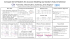### Dynamic Intelligent Kernel Assignment in Heterogeneous MultiGPU Systems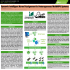### M. J. Ferrarotti, S. Decherchi and W. Rocchia CONCEPT Lab, Istituto### Bootchart 2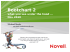### p - [email protected]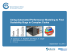### MandrakeLinux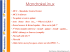### Visual Aid - UC Food Safety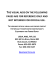### Lab 2 – Operating Systems CSCI 6303 – Principles of I.T.